# I.E. Irodov Solutions on Photometry And Geometrical Optics

Students can find the solutions of the Problems In General Physics I.E. Irodov - Photometry And Geometrical Optics on this page. Photometry and geometrical optics is an important topic in optics. The branch of physics that deals with the behavior and the properties of light is called optics. The important topics include luminous intensity and illuminance, illuminance produced by a point isotropic source, luminosity, and luminance, the relation between the refractive angle of a prism and least deviation angle, equation of spherical mirror, equations for an aligned optical system, relations between focal lengths and optical power, optical power of a spherical refractive surface, etc.

Learning these solutions will help students to achieve higher marks in the JEE exam. Students are recommended to go through these solutions thoroughly before the exams to score well and improve their problem-solving abilities.

### I.E. Irodov Solutions on Photometry And Geometrical Optics

1. Making use of the spectral response curve for an eye (see Fig. 5.1), find:

(a) the energy flux corresponding to the luminous flux of 1.0 lm at the wavelengths 0.51 and 0.64 pm;

(b) the luminous flux corresponding to the wavelength interval from 0.58 to 0.63 tim if the respective energy flux, equal to 01), 4.5 mW, is uniformly distributed over all wavelengths of the interval. The function V (λ) is assumed to be linear in the given spectral interval.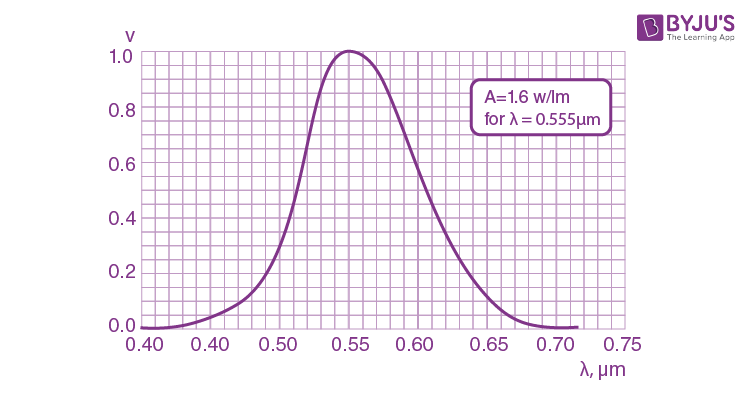Solution:

1. (a) The relative spectral response V(λ) shown in Fig. (5.11) of the book is so defined that A/V (λ) is the energy flux of light of wavelength λ, needed to produce a unit luminous flux at that wavelength. (A is the conversion factor defined in the book.)

At λ = 0 . 51 μm, we read

V(λ) = 0.17

And energy flux corresponding to a luminous flux of 1 lumen = 1.6/0.17

= 9.4 mW

(b) here dϕe(λ) = ϕe/(λ2 - λ1) dλ, λ1 ≤ λ ≤λ2

Since energy is distributed uniformly. Then

$\phi =\int_{\lambda _{1}}^{\lambda _{2}}V(\lambda )d\phi _{2}( \frac{\lambda}{A})$

= $\frac{\phi _{e}}{A(\lambda _{2}-\lambda _{1})}\int_{\lambda _{1}}^{\lambda _{2}}V(\lambda )d\lambda$

Since V (λ) is assumed to vary linearly in the interval λ1 ≤ λ ≤λ2,

we have

$\frac{1}{(\lambda _{1}-\lambda _{2})}\int_{\lambda _{1}}^{\lambda _{2}}V(\lambda )d\lambda =\frac{1}{2}(V(\lambda _{1})+V(\lambda _{2}))$

Thus ϕ = (ϕe/2A) (V(λ1)+ V(λ2))

Using V(0.58 μm) = 0.85

V(0.63 μm) = 0.25

Thus ϕ = (ϕe/2×1.6)×1.1 = 1.55 lumen

2. A point isotropic source emits a luminous flux (ϕ = 10 lm with wavelength λ = 0.59 μm. Find the peak strength values of electric and magnetic fields in the luminous flux at a distance r = 1.0 m from the source. Make use of the curve illustrated in Fig. 5.1.

Solution:

1. We have ϕe = ϕA/V(λ)

But ϕe = ½ √(ε00)Em2×4πr2/area

Or Em2 = (ϕA/2πr2V(λ))√(μ00)

For λ = 0.59 μm

V(λ) = 0.74

Thus Em = 1/14 V/m

Also Hm = √(ε00)Em

= 3.02 mA/m

3. Find the mean illuminance of the irradiated part of an opaque sphere receiving

(a) a parallel luminous flux resulting in illuminance E0 at the point of normal incidence;

(b) light from a point isotropic source located at a distance l = 100 cm from the centre of the sphere; the radius of the sphere is R = 60 cm and the luminous intensity is I = 36 cd.

Solution:

1. (a) Mean illuminance = Total luminous flux incident/Total area illuminated

Now, to calculate the total luminous flux incident on the sphere, we note that the illuminance at the point of normal incidence is E0. Thus the incident flux is E0πR2.

Mean illuminance = E0πR2/2πR2

E = ½ E0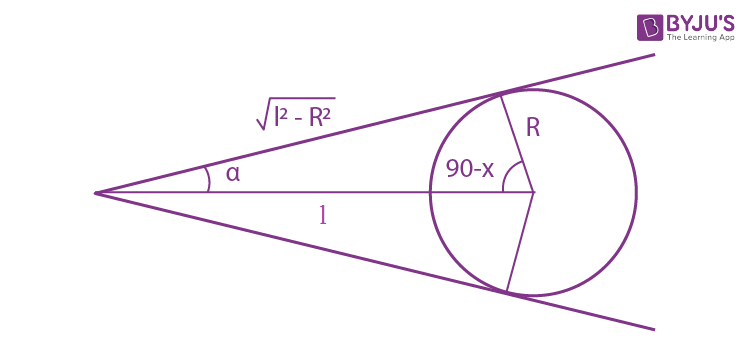(b) The sphere subtends a solid angle

2π(1 - cos α) = 2π(1 - √(l2 - R2)/l)

At a point source and therefore receives a total flux of 2πI(1 - √(l2 - R2)/l)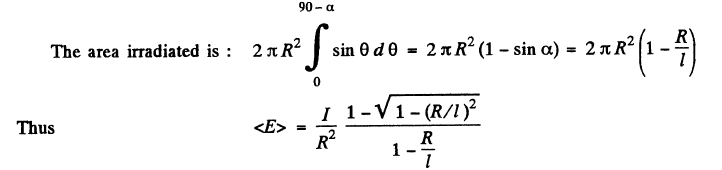Substituting we get E = 50 lux.

4. Determine the luminosity of a surface whose luminance depends on direction as L = L0 cos θ, where θ is the angle between the radiation direction and the normal to the surface.

Solution:

1. Luminance L is the light energy emitted per unit area of the emitting surface in a given direction per unit solid angle divided by cos θ. Luminosity M is simply energy emitted per unit area.

Thus M = ∫L. cos θ dΩ

where the integration must be in the forward hemisphere of the emitting surface (assuming light is being emitted in only one direction say the outward direction of the surface.)

But L = L0 cos θ

Thus M = ∫L0 cos2 θ . dΩ

= $2\pi \int_{0}^{\frac{\pi }{2}}L_{0}\cos ^{2}\theta \sin \theta d\theta =\frac{2}{3}\pi L_{0}$

5. A certain luminous surface obeys Lambert's law. Its luminance is equal to L. Find:

(a) the luminous flux emitted by an element ∆S of this surface into a cone whose axis is normal to the given element and whose aperture angle is equal to θ;

(b) the luminosity of such a source.

Solution:

1. For a Lambert source L = Constant

The flux emitted into the cone is

ϕ = L∆S cos α dΩ

= $L\Delta S\int_{0}^{\theta }2\pi \cos \alpha \sin \alpha d\alpha$

= L∆S π(1 - cos2θ)

= πL∆S sin2θ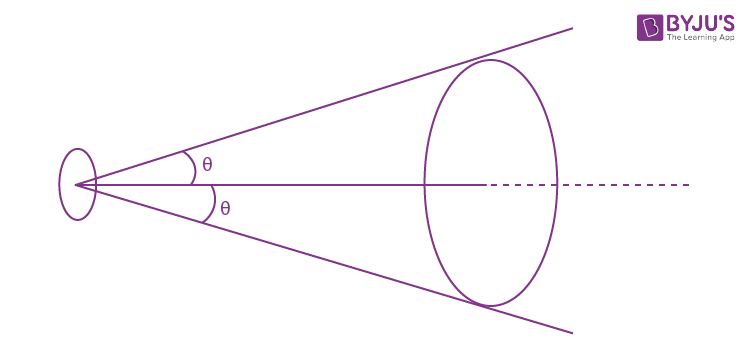(b) The luminosity is obtained from the previous formula for θ = 900

M = ϕ(θ = 900)/∆S = πL

6. An illuminant shaped as a plane horizontal disc S = 100 cm2 in area is suspended over the centre of a round table of radius R = 1.0 m. Its luminance does not depend on direction and is equal to L = 1.6×104 cd/m2. At what height over the table should the illuminant be suspended to provide maximum illuminance at the circumference of the table? How great will that illuminance be? The illuminant is assumed to be a point source.

Solution:

1. The equivalent luminous intensity in the direction OP is LS cos θ and the illuminance at P is

[(LS cos θ)/(R2 + h2)] cos θ = LSh2/(R2 + h2)2

= LS/[(R/√h - √h)2 + 2R]2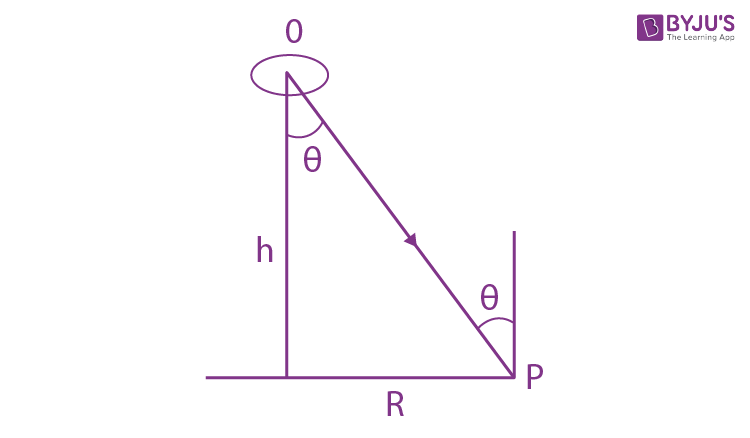This is maximum when R = h and the maximum illuminance is

LS/4R2 = 1.6×102/4

= 40 lux

7. A point source is suspended at a height h = 1.0 m over the centre of a round table of radius R = 1.0 m. The luminous intensity I of the source depends on direction so that illuminance at all points of the table is the same. Find the function I (θ), where θ is the angle between the radiation direction and the vertical, as well as the luminous flux reaching the table if I(0) = I0 = 100 cd.

Solution:

1. The illuminance at P is

Ep = I(θ) cos θ/(x2 + h2)

= I(θ) cos3 θ/ h2

Since this is constant at all x, we must have

I(θ) cos3 θ = constant = I0

Or I(θ) = I0/cos3θ

The luminous flux reaching the table is

ϕ = πR2 I0/h2

= 314 lumen.

8. A vertical shaft of light from a projector forms a light spot S = 100 cm2 in area on the ceiling of a round room of radius R = 2.0 m. The illuminance of the spot is equal to E = 1000 lx. The reflection coefficient of the ceiling is equal to ρ = 0.80. Find the maximum illuminance of the wall produced by the light reflected from the ceiling. The reflection is assumed to obey Lambert's law.

Solution:

1. The illuminated area acts as a Lambert source of luminosity M = πL where

MS = ρES = total reflected light

Thus the luminance, L = ρE/π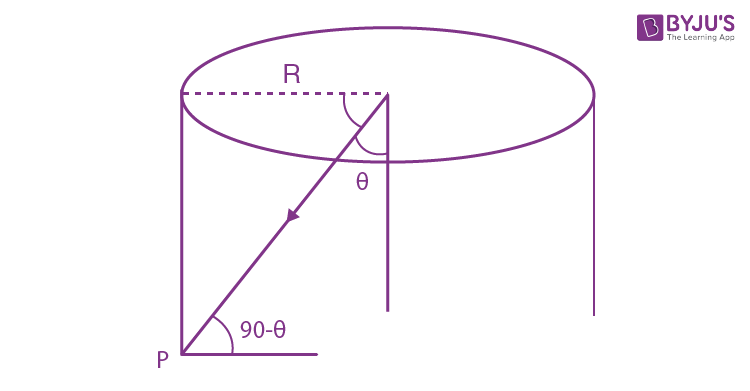The equivalent luminous intensity in the direction making an angle θ from the vertical is

LS cos θ = ρES cos θ/π

and the illuminance at the point P is

(ρES/π)cos θ sin θ/R2 cosec2 θ = (ρES/πR2)cos θ sin3 θ

This is maximum when (d/dθ)(cos θ sin3 θ) = -sin4θ + 3 sin2θ cos2θ = 0

Or tan2 θ = 3

⇒ tan θ = √3

Then the maximum illuminance is

(3√3/16π)ρES/R2

This illuminance is obtained at a distance R cot θ = R/√3 from the ceiling. Substitution gives the value 0.21 lux.

9. A luminous dome-shaped as a hemisphere rests on a horizontal plane. Its luminosity is uniform. Determine the illuminance at the centre of that plane if its luminance equals L and is independent of direction.

Solution:

1.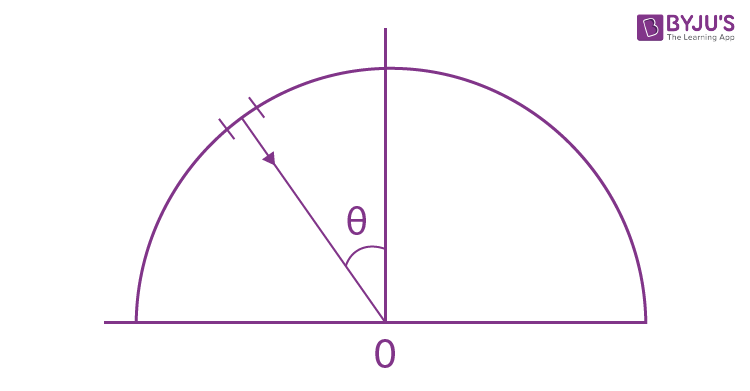From the definition of luminance, the energy emitted in the radial direction by an element dS of the surface of the dome is

dϕ = L dS d Ω

Here L = constant. The solid angle dΩ is given by

dΩ = dA cos θ/R2

where dA is the area of an element on the plane illuminated by the radial light. Then

dϕ = (L dS dA/R2)cos θ

The illuminance at 0 is then

E = ∫ dϕ/dA = $\int_{0}^{\frac{\pi }{2} }\frac{L}{R^{2}}2\pi R^{2}\sin \theta d\theta \cos \theta$

= $2\pi L\int_{0}^{l} xdx$

= πL

10. A Lambert source has the form of an infinite plane. Its luminance is equal to L. Find the illuminance of an area element-oriented parallel to the given source.

Solution:

1.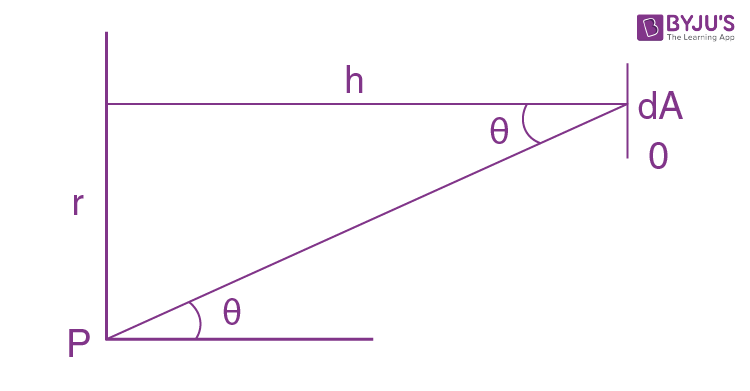Consider an element of area dS at point P.

It emits light of flux

dϕ = L dS dΩ cos θ

= L dS (dA/h2 sec2 θ)cos2θ

= (L dS dA/h2)cos4θ

In the direction of the surface element dA at O.

The total illuminance at O is then

E = ∫(LdS/h2) cos4θ

But dS= 2πr dr = 2πh tan θ d(h tan θ)

= 2πh2 sec2 θ tan θ dθ

Substitution gives

E = $2\pi L\int_{0}^{\frac{\pi }{2}}\sin \theta \cos \theta d\theta =\pi L$

11. An illuminant shaped as a plane horizontal disc of radius R = 25 cm is suspended over a table at a height h = 75 cm. The illuminance of the table below the centre of the illuminant is equal to E0 = 70 lx. Assuming the source to obey Lambert's law, find its luminosity.

Solution:

1.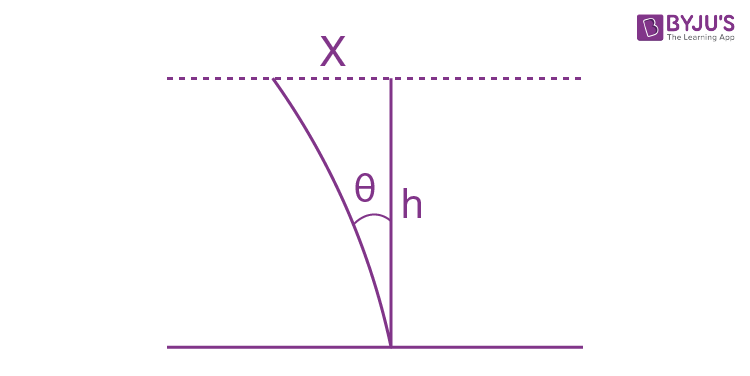Consider an angular element of area

2πx dx = 2πh2 tan θ sec2 θ dθ

Light emitted from this ring is

dϕ = L dΩ(2πh2 tan θ sec2 θ dθ) cos θ

Now dΩ = dA cos θ/h2 sec2 θ

Where dA = an element of area of the table just below the centre of the illuminant.

Then the illuminance at the element dA will be

E0 = $\int_{\theta =0}^{\theta =\alpha }2\pi L\sin \theta \cos \theta d\theta$

where sin α = R/√(h2 + R2).

Finally using luminosity M = πL

E0 = M sin2 α = M R2/(h2 + R2)

Or M = E0(1 + h2/R2)

= 700 lm/m2

(1 lx = 1 lm/m2 dimensionally).

12. A small lamp having the form of a uniformly luminous sphere of radius R = 6.0 cm is suspended at a height h = 3.0 m above the floor. The luminance of the lamp is equal to L = 2.0×104 cd/m2 and is independent of direction. Find the illuminance of the floor directly below the lamp.

Solution:

1. See the figure below.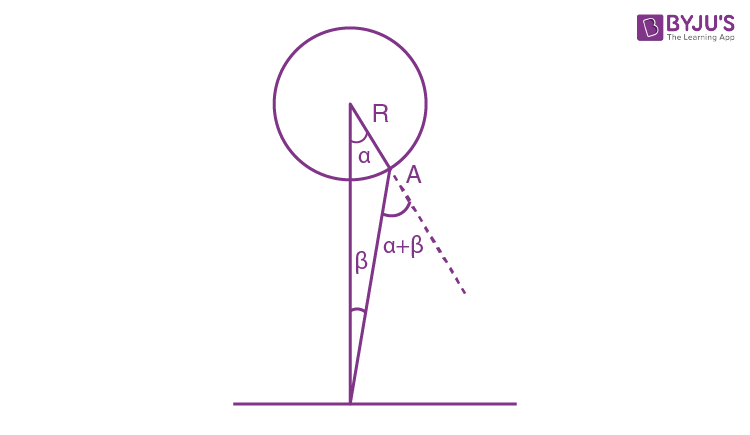The light emitted by an element of the illuminant towards the point 0 under consideration is

dϕ = L dS dΩ cos (α + β)

The element dS has the area

dS = 2πR2 sin α dα

The distance OA = [ h2 + R2 - 2hR cos α]1/2

We also have OA/sin α = h/sin(α + β) = R/sin β

From the diagram

cos (α + β) = (h cos α - R)/OA

cos β = (h - R cos α)/OA

If we imagine a small area d∑ at O, then

d∑cos β(/OA)2 = dΩ

Hence the illuminance at O is

∫ dϕ/d∑ = ∫ L 2πR2 sin α dα(h cos α - R) (h - R cos α)/(OA)4

The limit of α is α = 0 to that value for which α + β = 900, for then light is emitted tangentially. Thus αmax = cos-1 (R/h)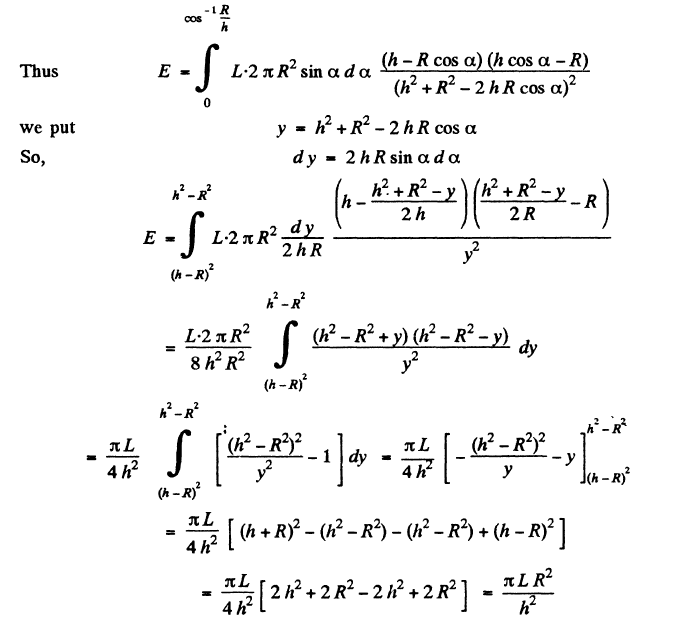Substitution gives: E = 25.1 lux.

13. Write the law of reflection of a light beam from a mirror in vector form, using the directing unit vectors e and e’ of the incident and reflected beams and the unit vector n of the outside normal to the mirror surface.

Solution:

1. We see from the diagram that because of the law of reflection, the component of the incident unit vector e along vector n changes sign on reflection while the component || to the mirror remains unchanged.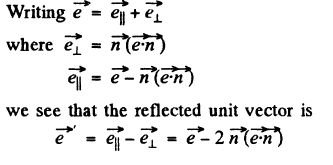14. Demonstrate that a light beam reflected from three mutually perpendicular plane mirrors in succession reverses its direction.

Solution:

1. We choose the unit vectors perpendicular to the mirror as the x, y, z axes in space. Then after reflection from the mirror with normal along x axis

$\vec{e'}=-e_{x}\hat{i}+e_{y}\hat{j}+e_{z}\hat{k}$

where $\hat{i}, \hat{j}, \hat{k}$ are the basic unit vectors. After a second reflection from the 2nd mirror say along y axis.

$\vec{e''}=-e_{x}\hat{i}-e_{y}\hat{j}+e_{z}\hat{k}$

Finally after the third reflection

$\vec{e'''}=-e_{x}\hat{i}-e_{y}\hat{j}-e_{z}\hat{k}=-\vec{e}$

15. At what value of the angle of incident θ1 is a shaft of light reflected from the surface of water perpendicular to the refracted shaft?

Solution:

1.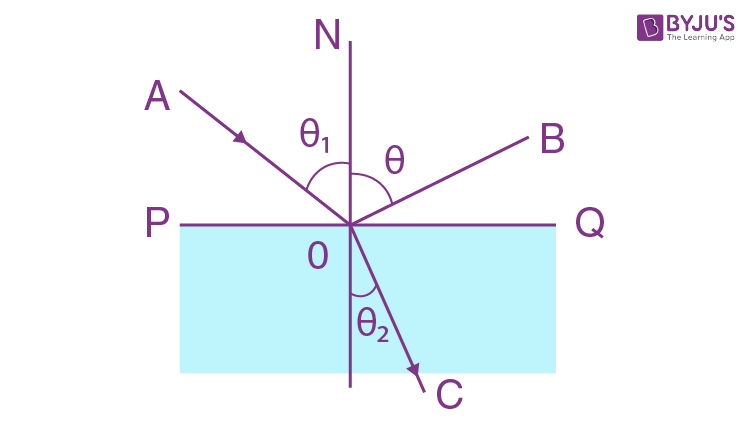Let PQ be the surface of water and n be the R.I. of water. Let AO is the shaft of light with incident angle θ1 and OB and OC are the reflected and refracted light rays at angles θ1 and θ2 respectively(Fig). From the figure θ2 = π/2 - θ1

From the law of reflection at the interface PQ

n = sin θ1/sin θ2 = sin θ1/sin (π/2 - θ1)

Or n = sin θ1/cos θ1 = tan θ1

Hence θ1 = tan-1 n.

16. Two optical media have a plane boundary between them. Suppose θ1cr is the critical angle of incidence of a beam and θ1 is the angle of incidence at which the refracted beam is perpendicular to the reflected one (the beam is assumed to come from an optically denser medium). Find the relative refractive index of these media if sin θ1cr/sin θ1 = η = 1.28.

Solution:

1.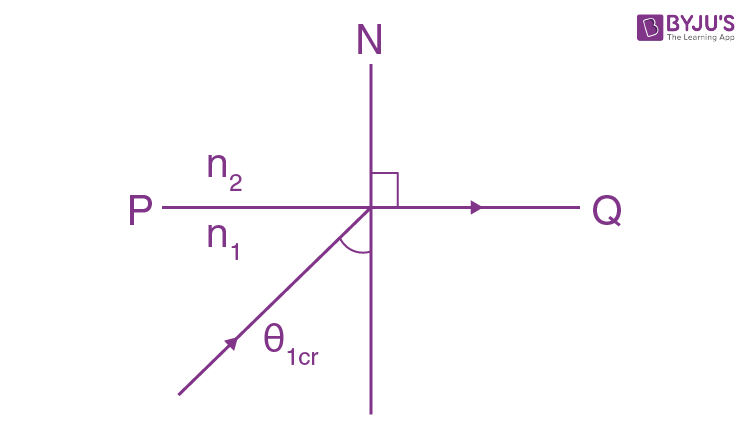Let two optical mediums of R.I. n1 and n2 respectively be such that n1 >n2. In the case when angle of incidence is θ1cr (Fig.), from the law of refraction

n1 sin θ1cr = n2 (1)

In the case, when the angle of incidence is θ1, from the law of refraction at the interface of mediums 1 and 2.

n1 sin θ1 = n2 sin θ2

But in accordance with the problem θ2 = (π/2 - θ1)

So n1sin θ1 = n2 cos θ1 (2)

Divide Eqn (1) by (2)

sin θ1cr/sin θ1 = 1/cos θ1

Or η = 1/cos θ1

So, cos θ1 = 1/η and sin θ1 = √(η2 - 1)/η (3)

But n1/n2 = cos θ1/sin θ1

So n1/n2 = (1/η)(η/√(η2 - 1) (using 3)

Thus n1/n2 = 1/√(η2 - 1)

17. A light beam falls upon a plane-parallel glass plate d = 6.0 cm in thickness. The angle of incidence is θ = 600. Find the value of deflection of the beam which passed through that plate.

Solution:

1.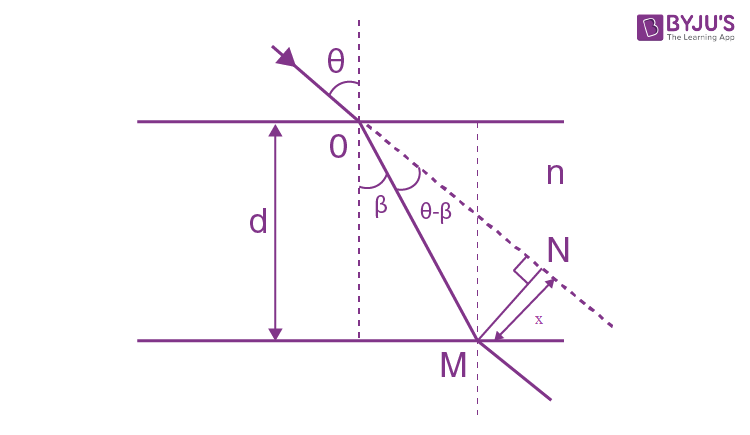From the fig. the sought lateral shift

x = OM sin (θ - β)

= d sec β sin (θ - β)

= d sec β (sin θ cos β - cos θ sin β)

= d(sin θ - cos θ tan β) (1)

But from the law of refraction

sin θ = n sin β or sin β = sin θ/n

So cos β = √(n2 - sin2θ)/n and tan β = sin θ/√(n2 - sin2θ)

Thus x = d (sin θ - cos θ tan β)

= d(sin θ - cos θ sin θ/√(n2 - sin2θ))

= d sin θ(1 - √(1 - sin2θ)/n2 - sin2θ)

18. A man standing on the edge of a swimming pool looks at a stone lying on the bottom. The depth of the swimming pool is equal to h. At what distance from the surface of water is the image of the stone formed if the line of vision makes an angle θ with the normal to the surface?

Solution:

1.From the fig.

sin dα = MP/OM = MN cos α/h sec α(α + dα)

As dα is very small, so

dα ≅ MN cos α/h sec α = MN cos2α/h (1)

Similarly

dθ = Mn cos2θ/h’ (2)

From Eqns (1) and (2)

dα/dθ = h’ cos2α/h cos2θ

Or h’ = (h cos2 θ/cos2α) dα/dθ (3)

From the law of refraction

n sin α = sin θ (A)

sin α = sin θ/n

So cos α = √(n2 - sin2θ)/n2 (B)

Differentiating Eqn. (A)

n cos α dα = cos θ dθ

Or dα/dθ = cos c/n cos α (4)

Using (4) in (3), we get

h’= h cos3θ/n cos3α (5)

Hence h’ = h cos3θ/n[(n2 - sin2θ)/n2]3/2

= n2h cos3θ/(n2 - sin2 θ)3/2 (using Eqn. (B))

19. Demonstrate that in a prism with small refracting angle θ the shaft of light deviates through the angle α ≈ (n- 1)θ regardless of the angle of incidence, provided that the latter is also small.

Solution:

1.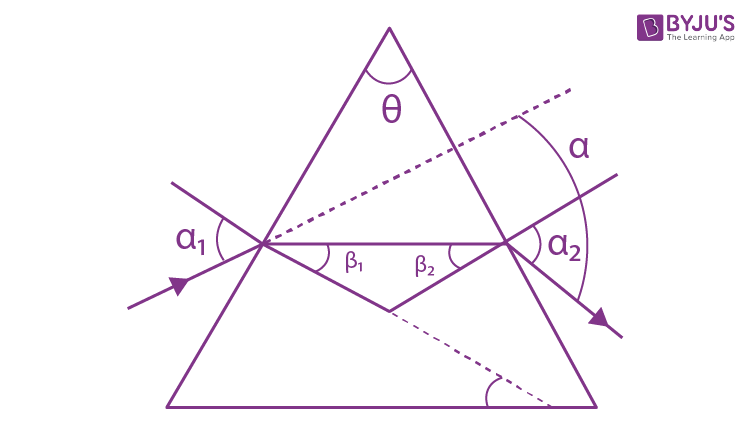The figure shows the passage of a monochromatic ray through the given prism, placed in air medium. From the figure, we have

θ = β1 + β2 (A)

And α = (α1 + α2) - (β1 + β2)

α = (α1 + α2) - θ (1)

From the Snell’s law,

sin α1 = n sin β1

Or α1 = nβ1 (for small angles) (2)

And sin α2 = n sin β2

Or α2 = nβ2 (for small angles) (3)

From Eqns (1), (2) and (3), we get

α = n( β1 + β2) - θ

So α = n(θ) - θ

= (n - 1)θ (using Eqn. A)

20. The least deflection angle of a certain glass prism is equal to its refracting angle. Find the latter.

Solution:

1. In this case we have

sin (α + θ)/2 = n sin θ/2

In our problem α = θ

So, sin θ = n sin (θ/2) or 2 sin (θ/2) cos (θ/2) = n sin (θ/2)

Hence cos (θ/2) = n/2

Or θ = 2 cos-1 (n/2)

= 830, where n = 1.5